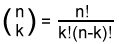The SDL Component Suite is an industry leading collection of components supporting scientific and engineering computing. Please visit the SDL Web site for more information....## BinomCoeff

 Unit: SDL_statis Class: none Declaration: function BinomCoeff (n,k: integer): longint;

The function BinomCoeff calculates the binomial coefficient (n,k), which is the number of possible combinations when drawing k items out of a total set of n. The binomial coefficient is defined by the following formula:The parameters n, and k have to be positive, with n >= k. If this condition is not fulfilled, BinomCoeff returns a zero value.

Please note that the binomial coefficient may become too large to be returned as longint value. In this case an EInvalidOp exception is generated. If you expect large binomial coefficients you maybe want to use the function LnBinomCoeff, which calculates the natural logarithm of it.

Last Update: 2018-Oct-06